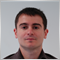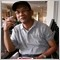How to create a variable to point to double &close[] ?312

int OnCalculate(const int rates_total,       // number of available bars in history at the current tick
const int prev_calculated,   // number of bars, calculated at previous tick
const datetime &time[],
const double &open[],
const double &high[],
const double &low[],
const double &close[],
const long &tick_volume[],
const long &volume[],
const int &spread[])   {

double yy[];
yy = close[]; // error here, "invalid array access"
// question: how to create a variable to point to a reference to dynamic double array?

// so that the yy can be used in the following way:
// yy, yy, ....

}48716

i think you need to copy close[] to yy[]53986

nicolasxu:
double yy[];
//yy = close[]; // error occurs because the variable yy is not invoked as arrays, whereas yy is declared as an array (double yy[];)
//should:
yy=close;
yy=close; // etc312

3rjfx:
double yy[];
//yy = close[]; // error occurs because the variable yy is not invoked as arrays, whereas yy is declared as an array (double yy[];)
//should:
yy=close;
yy=close; // etc
I see. Thanks!